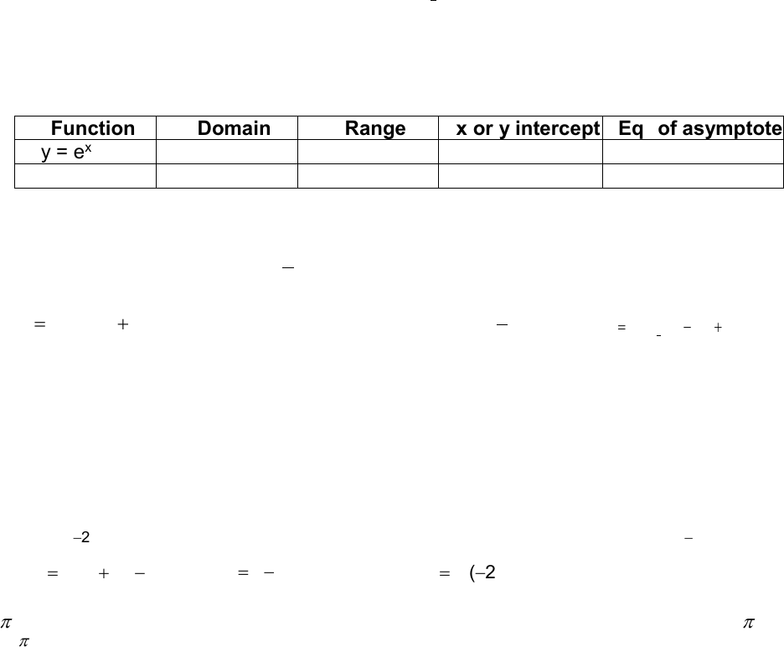Study Guides (390,000)
CA (150,000)
Western (10,000)
ENGSCI (30)
Midterm

# Engineering Science 1036A/B Midterm: 3.4 Graphing Exponential and Logarithmic Functions with Different BasesExam

Department
Engineering Science
Course Code
ENGSCI 1036A/B
Professor
Quazi Rahman
Study Guide
Midterm

This preview shows page 1. to view the full 4 pages of the document.Graphing Exponential and Logarithmic Functions with Different Bases
1. Sketch each function and its inverse.
a) y = 2x b) y = (0.5)x c) y =
x
4
1
log
d) y = log x
2. a) State the equation of the inverse of y = ex and sketch both functions on the same
grid.
b) Complete the following chart.
Function
Domain
Range
x or y intercept
Eqn of asymptote
y = ex
y = ln x
3. Use transformations to sketch graphs of these functions and state the domain and range.
a) y =2(4x)+1 b)
63
4
1+
=
x
y
c) y = 4-x 2 d) y = -(4)x + 1
e)
)2(log3 2+= xy
f) y = log 2x g)
h)
y x
= +
log ( )
1
2
3 1
4. For the curve
x
y
=)3(2
+ 5, determine
a) the equation of the horizontal asymptote
b) the y intercept
c) whether the curve is increasing or decreasing
d) the domain and range
5. Use transformations to draw the graph of each function and state its domain and range.
a)
x
ey 2
=
b)
3
22 = +x
ey
c)
x
ey 41=
d)
2
3
=x
ey
e)
1)2ln( += xy
f)
xy ln1=
g)
)2ln( xy =
6.
and e are both irrational numbers. Using a calculator, determine which is larger,
e or
e
?